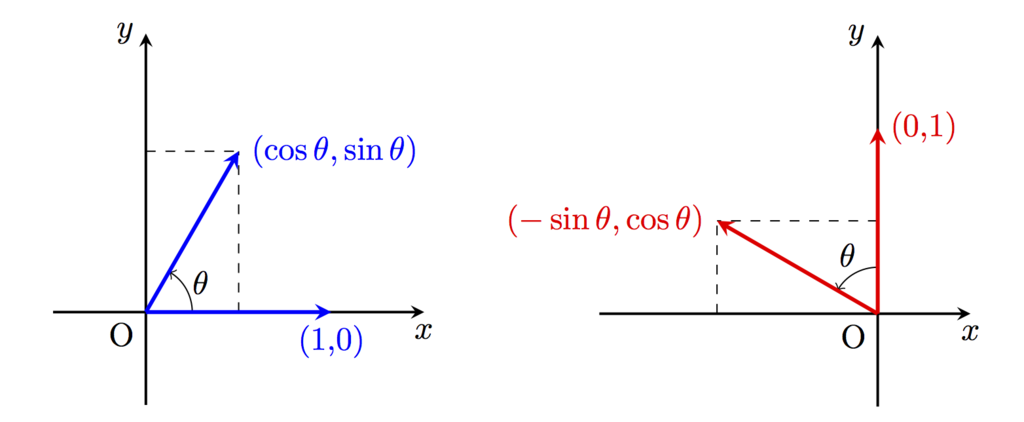Notes_EN

# Easy way to derive rotation matrix

It is very easy to derive rotation matrix.
This method is applied to general cases.

### Derivation

#### Step1. Calculate rotation of unit vectors

Calculate rotation of unit vector of $x$ axis by $\theta$
\begin{align}
\left(
\begin{array}{c}
1\\
0
\end{array}
\right)
%
\overset{\text{Rotate by }\theta}{\longrightarrow}
%
\color{blue}{
\left(
\begin{array}{c}
\cos\theta\\
\sin\theta
\end{array}
\right)
}
\end{align}

Same way , rotate unit vector of $y$ axis
\begin{align}
\left(
\begin{array}{c}
0\\
1
\end{array}
\right)
%
\overset{\text{Rotate by }\theta}{\longrightarrow}
%
\color{red}{
\left(
\begin{array}{c}
-\sin\theta\\
\cos\theta
\end{array}
\right)
}
\end{align}#### Step2. Arrange two vectors

Just arrange these two vectors and we got Rotation Matrix!
\begin{align}
\left(
\begin{array}{cc}
\color{blue}{\cos\theta}&\color{red}{-\sin\theta}\\
\color{blue}{\sin\theta}&\color{red}{\cos\theta}
\end{array}
\right)
\end{align}

### Background: Why can we derive?

What we have to know is following simple property:

Useful property of matrix
Any matrix $M$ can be written as \begin{align} M=(Me_1,...,Me_n), \end{align} where \begin{align} e_i= \left( \begin{array}{c} 0 \\ \vdots \\ 1\\ \vdots \\ 0 \end{array} \right) (i~~~ \end{align} is a unit vector.

From this fact, we can derive any matrix by calculating transformation of each unit vectors.

Proof.
Let $(Me_i)_j$ be the $j$-th element of the vector $Me_i$.
Then $(Me_i)_j=\sum_{k=1}^n M_{jk}(e_i)_k=M_{ji}$.
Where, we use
\begin{align}
(e_i)_k=\delta_{ik}=
\begin{cases}
\,1& (k=i)\\
\,0& (k\neq i)
\end{cases}.
\end{align}
($\delta_{ik}$ is called Kronecker delta.)